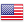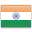Grade "A+" Accredited by NAAC with a CGPA of 3.46
Grade "A+" Accredited by NAAC with a CGPA of 3.46

# Digital Image Processing

Course ID
BHCS 16A
Level
Program
B.Sc. CS (Hons.)
Semester
Fifth
Credits
6.0
Paper Type
DSE - 2
Method
Lecture & Practical

## Unique Paper Code: Update Awaited

This course introduces students to the fundamentals of digital image processing, and various image transforms, image restoration techniques, image compression and segmentation used in digital image processing.

## Learning Outcomes:

At the end of the course, students should be able to:

• Describe the roles of image processing systems in a variety of applications;
• Write programs to read/write and manipulate images: enhancement, segmentation, and compression, spatial filtering.
• Develop Fourier transform for image processing in frequency domain.
• Evaluate the methodologies for image segmentation, restoration.

## Course Contents

Unit 1
Unit 2
Unit 3
Unit 4
Unit 5
Unit 6

Unit 1

Introduction: Digital Image Fundamentals: Brightness, Adaptation and Discrimination, Light and Electromagnetic Spectrum, Image Sampling and Quantization, Some Basic Relationships between Pixels Types of images.

Unit 2

Spatial Domain Filtering: Some Basic Intensity Transformation Functions, Histogram Equalization, Spatial Correlation and Convolution, Smoothening Spatial Filters: Low pass filters, Order Statistics filters; Sharpening Spatial Filters: Laplacian filter.

Unit 3

Filtering in Frequency Domain: The Discrete Fourier Transformation (DFT), Frequency Domain Filtering: Ideal and Butterworth Low pass and High pass filters, DCT Transform (1D, 2D).

Unit 4

Image Restoration: Image Degradation/Restoration Process, Noise models, Noise Restoration Filters
Image Compression: Fundamentals of Image Compression, Huffman Coding, Run Length Coding, JPEG.

Unit 5

Morphological Image Processing: Erosion, Dilation, Opening, Closing, Hit-or-Miss Transformation, Basic Morphological Algorithms.

Unit 6

Image Segmentation: Point, Line and Edge Detection, Thresholding, Region Based Segmentation.

### Practicals

#### Lab List 1

1. Write program to read and display digital image using MATLAB or SCILAB
2. a. Become familiar with SCILAB/MATLAB Basic commands.

b. Read and display image in SCILAB/MATLAB

c. Resize given image

d. Convert given color image into gray-scale image

e. Convert given color/gray-scale image into black & white image

f. Draw image profile

g. Separate color image in three R G & B planes

h. Create color image using R, G and B three separate planes

i. Flow control and LOOP in SCILAB

j. Write given 2-D data in image file

3. To write and execute image processing programs using point processing method
4. a. Obtain Negative image

b. Obtain Flip image

c. Thresholding

d. Contrast stretching.

5. To write and execute programs for image arithmetic operations
6. a. Addition of two images

b. Subtract one image from other image

c. Calculate mean value of image

d. Different Brightness by changing mean value

7. To write and execute programs for image logical operations
8. a. AND operation between two images

b. OR operation between two images

c. Calculate intersection of two images

d. Water Marking using EX-OR operation

e. NOT operation (Negative image)

9. To write a program for histogram calculation and equalization using
10. a. Standard MATLAB function

b. Program without using standard MATLAB functions

c. C Program

11. To write and execute program for geometric transformation of image
12. a. Translation

b. Scaling

c. Rotation

d. Shrinking

e. Zooming

13. To understand various image noise models and to write programs for
14. a. image restoration

b. Remove Salt and Pepper Noise

c. Minimize Gaussian noise

d. Median filter and Weiner filter

15. Write and execute programs to remove noise using spatial filters
16. a. Understand 1-D and 2-D convolution process

b. Use 3×3 Mask for low pass filter and high pass filter

17. Write and execute programs for image frequency domain filtering
18. a. Apply FFT on given image

b. Perform low pass and high pass filtering in frequency domain

c. Apply IFFT to reconstruct image

19. Write a program in C and MATLAB/SCILAB for edge detection using different edge detection mask

#### Lab List 2

1. Write and execute program for image morphological operations erosion and dilation.
2. To write and execute program for wavelet transform on given image and perform inverse wavelet transform to reconstruct image.

#### Text Books

Gonzalez, R. C., & Woods, R. E. (2017). Digital Image Processing. 4th edition. Pearson Education.
Jain, A. K. (1988). Fundamentals of Digital Image Processing. 1st edition Prentice Hall of India.

Castleman, K. R. (1995.). Digital Image Processing. 1st edition. Pearson Education
Gonzalez, R. C., Woods, R. E., & Eddins, S. (2004). Digital Image Processing using MATLAB. Pearson Education Inc.
Schalkoff, D. (1989). Image Processing and Computer Vision. 1st edition. John Wiley and Sons.

#### Assessment Methods

Written tests, assignments, quizzes, presentations as announced by the instructor in the class.

#### Teaching Learning Process

Use of ICT tools in conjunction with traditional class room teaching methods
Interactive sessions
Class discussions

#### Keywords

image transform, image restoration, image processing, image segmentation.

Disclaimer: Details on this page are subject to change as per University of Delhi guidelines. For latest update in this regard please refer to the University of Delhi website here.Englishहिन्दी